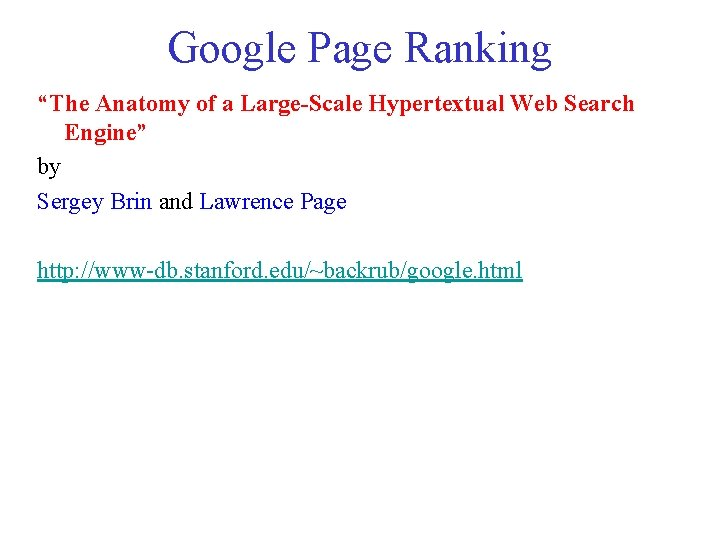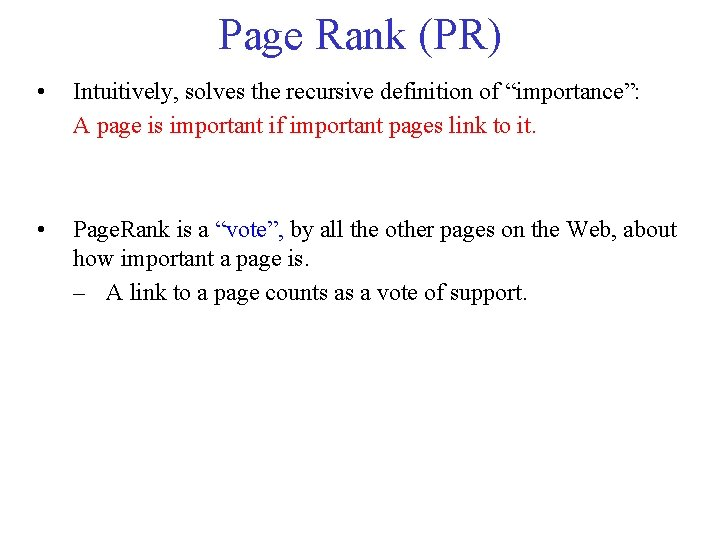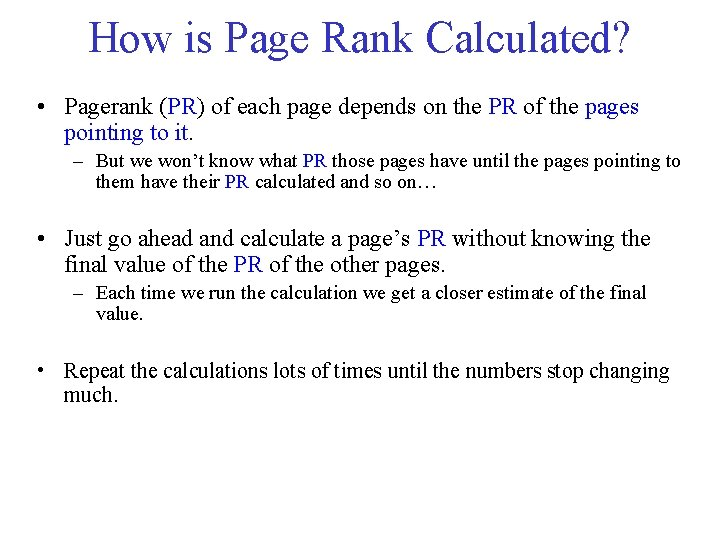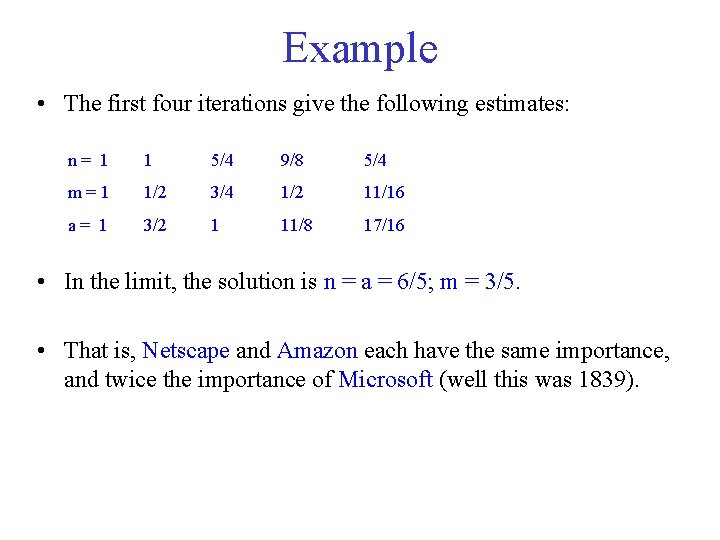• Slides: 11Google Page Ranking “The Anatomy of a Large-Scale Hypertextual Web Search Engine” by Sergey Brin and Lawrence Page http: //www-db. stanford. edu/~backrub/google. htmlPage Rank (PR) • Intuitively, solves the recursive definition of “importance”: A page is important if important pages link to it. • Page. Rank is a “vote”, by all the other pages on the Web, about how important a page is. – A link to a page counts as a vote of support.Page Rank Formula PR(A) = PR(T 1)/C(T 1) +…+ PR(Tn)/C(Tn) 1. T 1, . . . , Tn: pages that point (have outgoing links) to page A. 2. PR(Tn): current Pagerank value of Tn. Set to 1 initially. 3. C(Tn): count of outgoing links from page Tn. 4. PR(Tn)/C(Tn): portion of Pagerank value A gets from Tn. Each page spreads its vote out evenly amongst all of it’s outgoing links.How is Page Rank Calculated? • Pagerank (PR) of each page depends on the PR of the pages pointing to it. – But we won’t know what PR those pages have until the pages pointing to them have their PR calculated and so on… • Just go ahead and calculate a page’s PR without knowing the final value of the PR of the other pages. – Each time we run the calculation we get a closer estimate of the final value. • Repeat the calculations lots of times until the numbers stop changing much.Web Matrix Capture the formula by the web matrix (M) that is: • If page j has n successors (links), then: – M[i, j] =1/n if page i is one of these n successors of page j, and – 0 otherwise. • Then, the importance vector containing the rank of each page is calculated by: Ranknew = M • RankoldExample • In 1839, the Web consisted on only three pages: Netscape, Microsoft, and Amazon. For example, the first column of the Web matrix reflects the fact that Netscape divides its importance between itself and Amazon. The second column indicates that Microsoft gives all its importance to Amazon. Start with n = m = a = 1, then do rounds of improvements.Example • The first four iterations give the following estimates: n= 1 1 5/4 9/8 5/4 m=1 1/2 3/4 1/2 11/16 a= 1 3/2 1 11/8 17/16 • In the limit, the solution is n = a = 6/5; m = 3/5. • That is, Netscape and Amazon each have the same importance, and twice the importance of Microsoft (well this was 1839).Problems With Real Web Graphs Dead ends: a page that has no successors has nowhere to send its importance. Eventually, all importance will “leak out of” the Web. Example: Suppose Microsoft tries to claim that it is a monopoly by removing all links from its site. Eventually, each of n, m, and a become 0; i. e. , all the importance leaked out. n = 1 1 3/4 5/8 1/2 m = 1 1/2 1/4 3/16 a = 1 1/2 3/8 5/16Problems With Real Web Graphs Spider traps: a group of one or more pages that have no links out of the group will eventually accumulate all the importance of the Web. Example: Angered by the decision, Microsoft decides it will link only to itself from now on. Now, Microsoft has become a spider trap. Now, m converges to 3, and n = a = 0. n = 1 1 3/4 5/8 1/2 m = 1 3/2 7/4 2 35/16 a = 1 1/2 3/8 5/16Google Solution to Dead Ends and Spider Traps Stop the other pages having too much influence. This vote is “damped down” by multiplying it by a factor. Example: If we use a 20% damp-down, the equation of previous example becomes: The solution to this equation is n = 7/11; m = 21/11; a = 5/11.